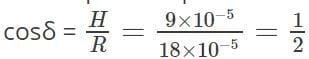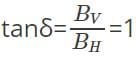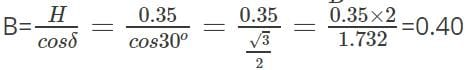NEET  >  Test: Earth's Magnetism

# Test: Earth's Magnetism

Test Description

## 10 Questions MCQ Test Physics Class 12 | Test: Earth's Magnetism

Test: Earth's Magnetism for NEET 2023 is part of Physics Class 12 preparation. The Test: Earth's Magnetism questions and answers have been prepared according to the NEET exam syllabus.The Test: Earth's Magnetism MCQs are made for NEET 2023 Exam. Find important definitions, questions, notes, meanings, examples, exercises, MCQs and online tests for Test: Earth's Magnetism below.
Solutions of Test: Earth's Magnetism questions in English are available as part of our Physics Class 12 for NEET & Test: Earth's Magnetism solutions in Hindi for Physics Class 12 course. Download more important topics, notes, lectures and mock test series for NEET Exam by signing up for free. Attempt Test: Earth's Magnetism | 10 questions in 10 minutes | Mock test for NEET preparation | Free important questions MCQ to study Physics Class 12 for NEET Exam | Download free PDF with solutions
 1 Crore+ students have signed up on EduRev. Have you?
Test: Earth's Magnetism - Question 1

### How many quantities are required to specify the magnetic field of the earth?

Detailed Solution for Test: Earth's Magnetism - Question 1

Three quantities are needed to specify the magnetic field of the earth on its surface, namely, the horizontal component, the magnetic declination and the magnetic dip. These are known as elements of the earth’s magnetic field or magnetic elements.

Test: Earth's Magnetism - Question 2

### Which of the following is the definition for magnetic meridian of Earth?

Detailed Solution for Test: Earth's Magnetism - Question 2

A vertical plane passing through the axis of a freely suspended or pivoted magnet is known as magnetic meridian of Earth. The vertical plane passing through the geographical North Pole and South Pole at a given place is known as the geographical meridian of that place.

Test: Earth's Magnetism - Question 3

### Which among the following is denoted by δ?

Detailed Solution for Test: Earth's Magnetism - Question 3

Magnetic dip or inclination at a place is defined as the angle made by the Earth’s magnetic field with the horizontal in the magnetic meridian. It is denoted by δ.
Magnetic declination at a place is defined as the angle between the geographic meridian and magnetic meridian.

Test: Earth's Magnetism - Question 4

The Earth always have both horizontal and vertical components everywhere.

Detailed Solution for Test: Earth's Magnetism - Question 4

No, this statement is false. The Earth always has a vertical component except at the equator. Similarly, the earth always has a horizontal component except at the poles.

Test: Earth's Magnetism - Question 5

Identify the expression for horizontal component from the following.

Detailed Solution for Test: Earth's Magnetism - Question 5

Horizontal component is a component of Earth’s magnetic field along the horizontal direction in the magnetic meridian. It is denoted by BH. If B is the intensity of Earth’s total magnetic field, then the horizontal component of Earth’s magnetic field is given by:
BH=Bcosδ

Test: Earth's Magnetism - Question 6

At the magnetic North Pole of the Earth, what is the value of the angle of dip?

Detailed Solution for Test: Earth's Magnetism - Question 6

Angle of dip is 90o at geographical North Pole because attraction on the North Pole of needle is very strong and the needle remains in vertical plane. At the magnetic equator, the needle will point horizontally, i.e. dip angle is 0o. As you move from the magnetic equator towards the magnetic pole, the angle increases in the northern hemisphere. So, the angle of dip is maximum.

Test: Earth's Magnetism - Question 7

At a given place on the Earth’s surface, the horizontal component of Earth’s magnetic field is 9 × 10-5 T and the resultant magnetic field is 180 × 10-6. Calculate the angle of dip at this place.

Detailed Solution for Test: Earth's Magnetism - Question 7

Given: H = 9 × 10-5; R = 180 × 10-6 = 18 × 10-5
The required equation ➔ H=RcosδTherefore, δ = cos-1 1/2

=60o
Thus, the angle of dip is 60o.

Test: Earth's Magnetism - Question 8

When is the angle of dip at a place equal to 45o?

Detailed Solution for Test: Earth's Magnetism - Question 8

Yes, the angle of dip at a place is equal to 45o, when the vertical and horizontal components of earth’s magnetic field are equal. In this case ➔ δ=45oSo, BV=BH.

Test: Earth's Magnetism - Question 9

The angle of dip at a certain place on Earth is 30o and the magnitude of Earth’s horizontal component of magnetic field is 0.35 G. Find the magnetic field at that place on Earth.

Detailed Solution for Test: Earth's Magnetism - Question 9

Given: Horizontal component (H) = 0.35 G; Angle of dip (δ) = 30o
Required equation ➔ cosδ= H/BTherefore, the magnitude of the magnetic field at that place is 0.40 G.

Test: Earth's Magnetism - Question 10

What is the relation between angle of dip and magnetic latitude?

Detailed Solution for Test: Earth's Magnetism - Question 10

If a magnet is freely suspended or inclined or dipped, the angle between the horizontal and the angle of inclination of the magnet that is inclined only by the earth’s magnetic field will indicate the magnetic latitude. The relation is as follows:
tanδ=2tanλ

## Physics Class 12

157 videos|452 docs|213 tests
Information about Test: Earth's Magnetism Page
In this test you can find the Exam questions for Test: Earth's Magnetism solved & explained in the simplest way possible. Besides giving Questions and answers for Test: Earth's Magnetism, EduRev gives you an ample number of Online tests for practice

## Physics Class 12

157 videos|452 docs|213 tests

### How to Prepare for NEET

Read our guide to prepare for NEET which is created by Toppers & the best Teachers(Scan QR code)